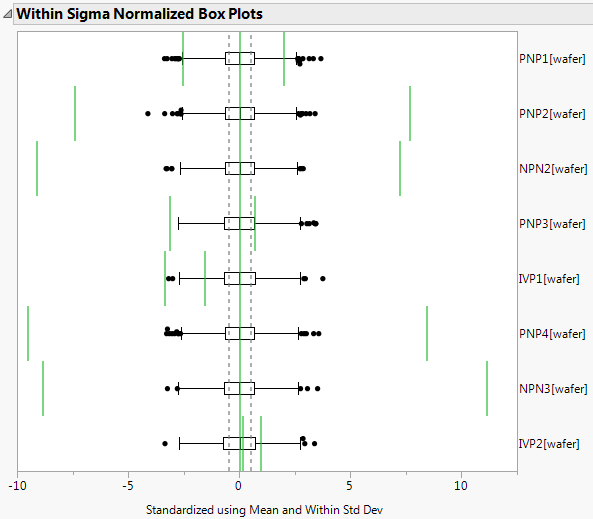Publication date: 05/24/2021

## Normalized Box Plots

The Normalized Box Plots options show or hide box plots that have been normalized using the specified sigma in the title. When drawing normalized box plots, JMP standardizes each column by subtracting the mean and dividing by the standard deviation. The box plots are formed for each column using these standardized values.

Figure 7.17 Within Sigma Normalized Box PlotFigure 7.17 shows the Within Sigma Normalized Box Plot for a selection of the process variables in the Semiconductor Capability.jmp sample data table using wafer as a subgroup variable.

The green vertical lines represent the specification limits for each variable normalized by the mean and standard deviation of each variable. The gray dotted vertical lines are drawn at ±0.5, since the data is standardized to a standard deviation of 1.